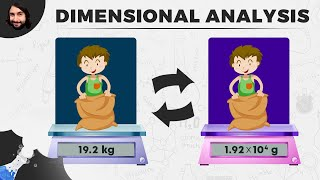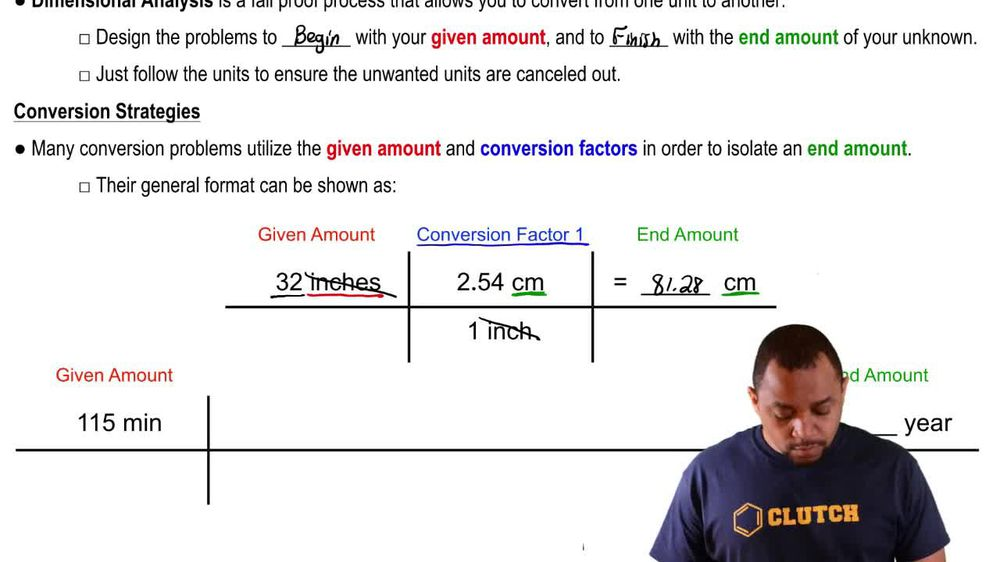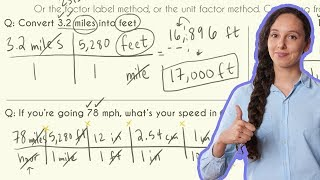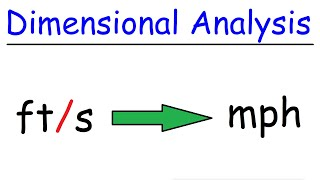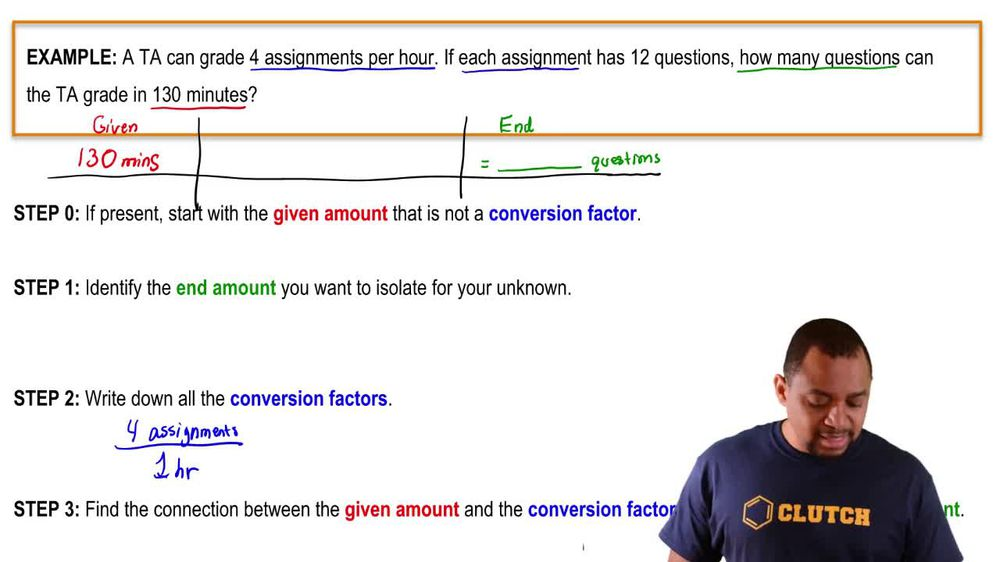Start typing, then use the up and down arrows to select an option from the list.
1. 1. Intro to General Chemistry2. Dimensional Analysis
Problem

# Perform the following conversions: (a) 5.00 days to s

Relevant Solution1m
Play a video:
Hi everyone, we have a question here, giving us the following units of time conversions, 60 seconds equals one minute, 60 minutes equals one hour, 24 hours equals one day, seven days equals one week. And it is asking us to calculate the number of seconds in a week and we have to give our answer with the correct number of significant figures. So it already gave us all the conversion factors we need to know. So we're just gonna start with our 60 seconds here And that equals one minute And then we're going to multiply by our 60 minutes And that is equal to one hour. So our minutes are canceling out here. Then we're going to multiply it by our 24 hours And that equals one day. Now our hours are canceling out here And then we're going to multiply that by seven days And that equals one week. So now our days are canceling out here and that is going to equal 6.0 times 10 to the fifth seconds. And we use two significant figures here because there are two significant figures in the problem. And that is our answer. Thank you for watching. Bye.